Documentation

### This is machine translation

Mouseover text to see original. Click the button below to return to the English version of the page.

To view all translated materials including this page, select Country from the country navigator on the bottom of this page.

## Wavelet Analysis for 3D Data

This example shows how to analyze 3D data using the three-dimensional wavelet analysis tool, and how to display low-pass and high-pass components along a given slice. The example focuses on magnetic resonance images.

A key feature of this analysis is to track the optimal, or at least a good, wavelet-based sparsity of the image which is the lowest percentage of transform coefficients sufficient for diagnostic-quality reconstruction.

To illustrate this, we keep the approximation of a 3D MRI to show the complexity reduction. The result can be improved if the images were transformed and reconstructed from the largest transform coefficients where the definition of the quality is assessed by medical specialists.

We will see that Wavelet transform for brain images allows efficient and accurate reconstructions involving only 5-10% of the coefficients.

### Load and Display 3D MRI Data

First, load the `wmri.mat` file which is built from the MRI data set that comes with MATLAB®.

`load wmri`

We now display some slices along the Z-orientation of the original brain data.

```map = pink(90); idxImages = 1:3:size(X,3); figure('DefaultAxesXTick',[],'DefaultAxesYTick',[],... 'DefaultAxesFontSize',8,'Color','w') colormap(map) for k = 1:9 j = idxImages(k); subplot(3,3,k); image(X(:,:,j)); xlabel(['Z = ' int2str(j)]); if k==2 title('Some slices along the Z-orientation of the original brain data'); end end```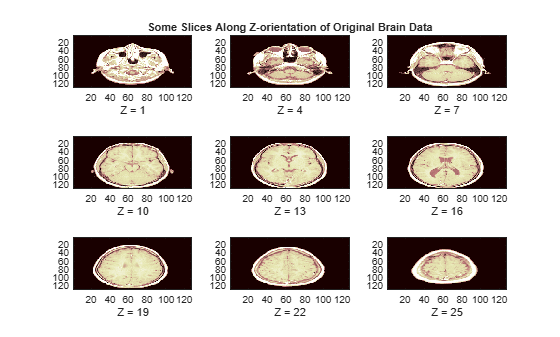We now switch to the Y-orientation slice.

```perm = [1 3 2]; XP = permute(X,perm); figure('DefaultAxesXTick',[],'DefaultAxesYTick',[],... 'DefaultAxesFontSize',8,'Color','w') colormap(map) for k = 1:9 j = idxImages(k); subplot(3,3,k); image(XP(:,:,j)); xlabel(['Y = ' int2str(j)]); if k==2 title('Some slices along the Y-orientation'); end end````clear XP`

### Multilevel 3D Wavelet Decomposition

Compute the wavelet decomposition of the 3D data at level 3.

```n = 3; % Decomposition Level w = 'sym4'; % Near symmetric wavelet WT = wavedec3(X,n,w); % Multilevel 3D wavelet decomposition.```

### Multilevel 3D Wavelet Reconstruction

Reconstruct from coefficients the approximations and details for levels 1 to 3.

```A = cell(1,n); D = cell(1,n); for k = 1:n A{k} = waverec3(WT,'a',k); % Approximations (low-pass components) D{k} = waverec3(WT,'d',k); % Details (high-pass components) end```

Check for perfect reconstruction.

```err = zeros(1,n); for k = 1:n E = double(X)-A{k}-D{k}; err(k) = max(abs(E(:))); end disp(err)```
``` 1.0e-09 * 0.3043 0.3043 0.3044 ```

### Display Low-Pass and High-Pass Components

The reconstructed approximations and details along the Z-orientation are displayed below.

```nbIMG = 6; idxImages_New = [1 7 10 16 19 25]; for ik = 1:nbIMG j = idxImages_New(ik); figure('DefaultAxesXTick',[],'DefaultAxesYTick',[],... 'DefaultAxesFontSize',8,'Color','w') colormap(map) for k = 1:n labstr = [int2str(k) ' - Z = ' int2str(j)]; subplot(2,n,k); image(A{k}(:,:,j)); xlabel(['A' labstr]); if k==2 title(['Approximations and details at level 1 to 3 - Slice = ' num2str(j)]); end subplot(2,n,k+n); imagesc(abs(D{k}(:,:,j))); xlabel(['D' labstr]); end end```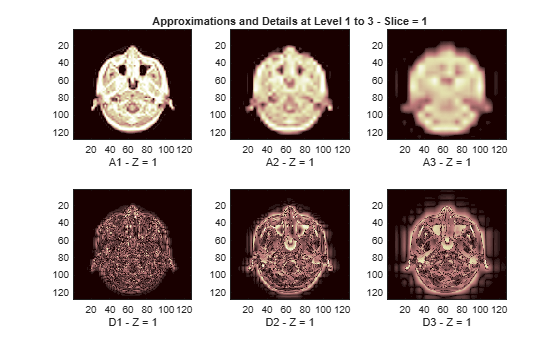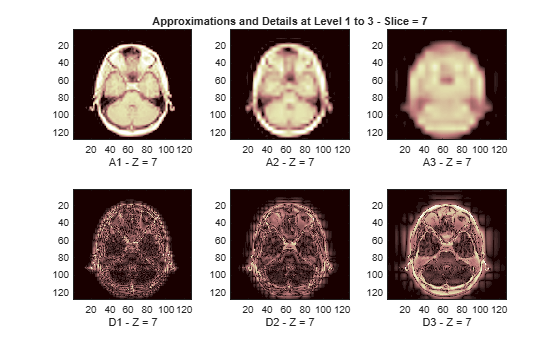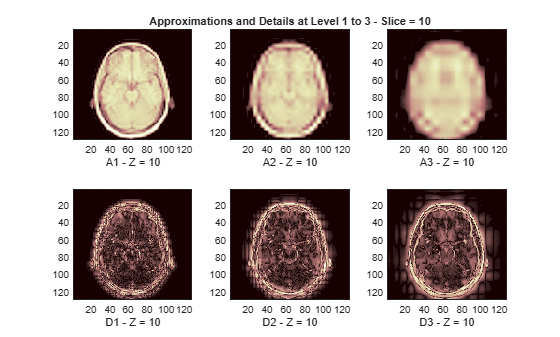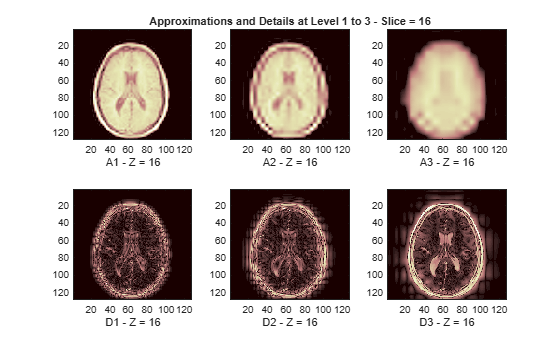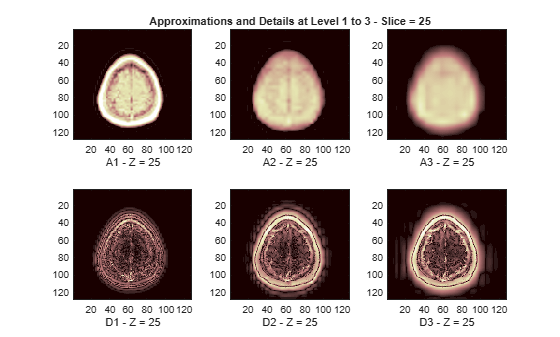### 3D Display of Original Data and Approximation at Level 2

The size of the 3D original array X is (128 x 128 x 27) = 442368. We can use a 3D display to show it.

```figure('DefaultAxesXTick',[],'DefaultAxesYTick',[],... 'DefaultAxesFontSize',8,'Color','w') XR = X; Ds = smooth3(XR); hiso = patch(isosurface(Ds,5),'FaceColor',[1,.75,.65],'EdgeColor','none'); hcap = patch(isocaps(XR,5),'FaceColor','interp','EdgeColor','none'); colormap(map) daspect(gca,[1,1,.4]) lightangle(305,30); fig = gcf; fig.Renderer = 'zbuffer'; lighting phong isonormals(Ds,hiso) hcap.AmbientStrength = .6; hiso.SpecularColorReflectance = 0; hiso.SpecularExponent = 50; ax = gca; ax.View = [215,30]; ax.Box = 'On'; axis tight title('Original Data');```The 3D array of the coefficients of approximation at level 2, whose size is (22 x 22 x 9) = 4356, is less than 1% the size of the original data. With these coefficients, we can reconstruct A2, the approximation at level 2, which is a kind of compression of the original 3D array. A2 can also be shown using a 3D display.

```figure('DefaultAxesXTick',[],'DefaultAxesYTick',[],... 'DefaultAxesFontSize',8,'Color','w') XR = A{2}; Ds = smooth3(XR); hiso = patch(isosurface(Ds,5),'FaceColor',[1,.75,.65],'EdgeColor','none'); hcap = patch(isocaps(XR,5),'FaceColor','interp','EdgeColor','none'); colormap(map) daspect(gca,[1,1,.4]) lightangle(305,30); fig = gcf; fig.Renderer = 'zbuffer'; lighting phong isonormals(Ds,hiso) hcap.AmbientStrength = .6; hiso.SpecularColorReflectance = 0; hiso.SpecularExponent = 50; ax = gca; ax.View = [215,30]; ax.Box = 'On'; axis tight title('Approximation at level 2');```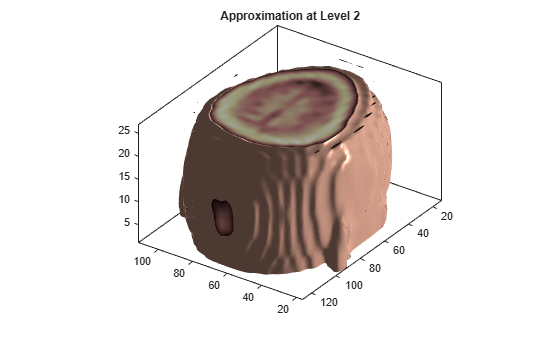### Summary

This example shows how to use 3D wavelet functions to analyze 3D data. The Wavelet Analyzer app, `waveletAnalyzer`, lets you perform the same steps more easily, such as simulating 3D visualization using an animation across different slices.

##### SupportGet trial now